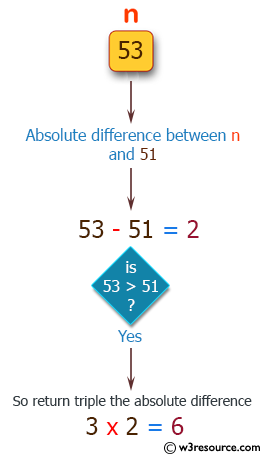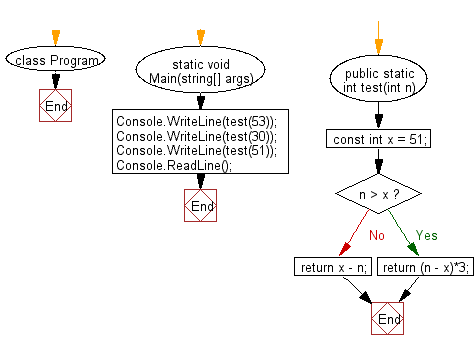﻿ C# - Get the absolute difference between n and 51# C# Sharp Basic Algorithm Exercises: Get the absolute difference between n and 51

## C# Sharp Basic Algorithm: Exercise-2 with Solution

Write a C# Sharp program to get the absolute difference between n and 51. If n is greater than 51 return triple the absolute difference.

Pictorial Presentation:Sample Solution:

C# Sharp Code:

``````using System;
namespace exercises
{
class Program
{
static void Main(string[] args)
{
Console.WriteLine(test(53));
Console.WriteLine(test(30));
Console.WriteLine(test(51));
}

public static int test(int n)
{
const int x = 51;

if (n > x)
{
return (n - x)*3;
}
return x - n;
}
}
}
```
```

Sample Output:

```6
21
0```

Flowchart:C# Sharp Code Editor:

Improve this sample solution and post your code through Disqus

What is the difficulty level of this exercise?

Test your Programming skills with w3resource's quiz.

﻿